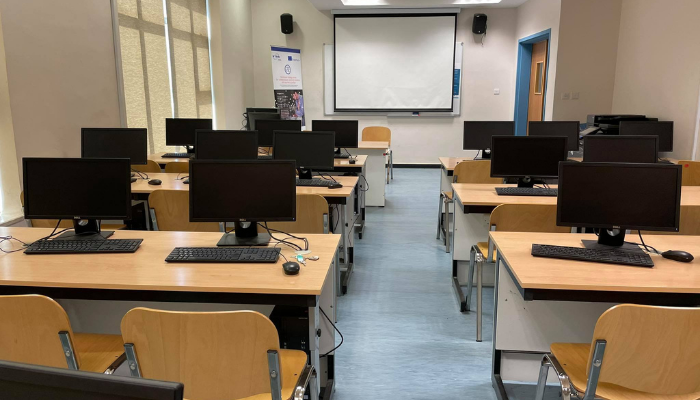﻿Course Catalog

1 Credit hour (3 hrs laboratory)

Introduce the concepts learned in the course through practical experiments using software application such as Matlab and Simulink. Topics covered include basics of different mathematical concepts (complex variables, Laplace transform, transfer function, zeros/ poles, and frequency domain plots), lumped/ distributed models of different physiological systems, control systems analysis and modeling in time domain and frequency domain, performance of first order, second order and higher order systems, block diagram, reduction of multiple subsystems, and root locus.

Lab Room:
M-327
Syllabus:acs_lab_outlines.pdf
Lab Supervisor: